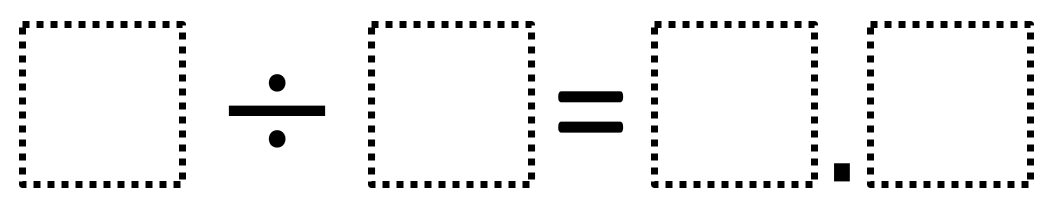# Dividing Whole Numbers With A Decimal Quotient

Directions: Using the digits 1 to 9 at most one time each, place a digit in each box to make a true statement.### Hint

How does choosing the divisor and dividend affect the quotient?
What digits would be bad choices for the divisor?
What digits would be bad choices for the dividend?

There are many possible answers including:
9 ÷ 2 = 4.5
6 ÷ 4 = 1.5
8 ÷ 5 = 1.6

Source: Owen Kaplinsky

## Add Fractions with Decimal Sums

Directions: Using the digits 1 to 9 at most one time each, place a digit …

1.5/3 = 1.7 (1.6 repeating)
9/5 = 1.8

2.In addition to the three solutions given by Owen and the second solution given by Mrs. Elliott there are five others: 1,2 = 6 ÷ 5, 1,4 = 7 ÷ 5, 1,5 = 3 ÷ 2, 1,5 = 9 ÷ 6 and 3,5 = 7 ÷ 2

3.4.9/2=4.5

5.6.7 divided by 2

7.7 divided by 2 =3.5

8.9 divided by 5 = 1.8

9.25 divided by 8=3.125